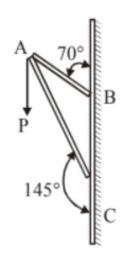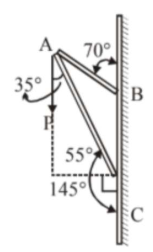# Consider a frame that is made up of two thin massless rods AB and AC as shown in the figure.Question:

Consider a frame that is made up of two thin massless rods $\mathrm{AB}$ and $\mathrm{AC}$ as shown in the figure. A vertical force $\overrightarrow{\mathrm{P}}$ of magnitude $100 \mathrm{~N}$ is applied at point $\mathrm{A}$ of the frame.Suppose the force is $\overrightarrow{\mathrm{P}}$ resolved parallel to the arms $\mathrm{AB}$ and $\mathrm{AC}$ of the frame. The magnitude of the resolved component along the arm $\mathrm{AC}$ is $\mathrm{xN}$.

The value of $\mathrm{x}$, to the nearest integer, is______

$\left[\right.$ Given : $\sin \left(35^{\circ}\right)=0.573, \cos \left(35^{\circ}\right)=0.819$

$\left.\sin \left(110^{\circ}\right)=0.939, \cos \left(110^{\circ}\right)=-0.342\right]$

Solution:Component along $\mathrm{AC}$

$=100 \cos 35^{\circ} \mathrm{N}$

$=100 \times 0.819 \mathrm{~N}$

$=81.9 \mathrm{~N}$

$\approx 82 \mathrm{~N}$